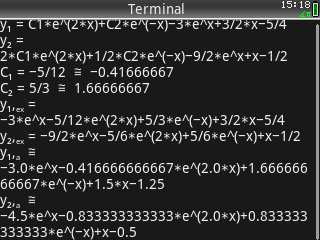## System of Two Linear Differential Equations

Description:Finds the solution to a system of two dífferential equations given as d(y1)/dx =*y1 + b*y2 + f(x) and d(y2)/dx =*y1 + d*y2 +g(x). For initial values x0, y1(x0), y2(x0) the constants of integration are calculated. A graph of both functions y1, y2 is provided.
Filename:systdiffequ.zip
ID:8941
Current version:1.01
Author:Claus Martin Dachselt
Size on calculator:4 KB
Platforms:Prime
User rating:Not yet rated (you must be logged in to vote)
Primary category:Math
Languages:ENG
File date:2018-10-24 10:05:33
Source code:Not included
Screenshot: